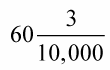##### Basic Math & Pre-Algebra For Dummies Book + Workbook BundleConverting decimals into fractions isn't hard. To convert a decimal into a fraction, you put the numbers to the right of the decimal point in the numerator (above the fraction line). Next, you place the number 1 in the denominator, and then add as many zeroes as the numerator has digits. Reduce the fraction if needed.

Decimals are nothing more than glorified fractions. They’re special because their denominators are always 10, 100, 1000, and so on — powers of 10. Watch where the decimal point is placed in the number. The number of decimal places to the right of the decimal point indicates the number of zeros in the power of 10 that is written in the denominator.

Example: Convert 3.025 to a fraction.

1. Check to see if there is a number before (to the left of) the decimal.

If there is, this number is the whole number of the fraction. So, 3 is the whole number.

2. Place the number that falls after (to the right of) the decimal in the numerator; then use the decimal placement to determine the denominator.

Place the number 1 in the denominator, and then add as many zeroes as the numerator has digits.

A digit is any single number from 0 through 9.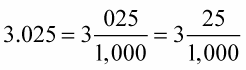3. Reduce the fraction.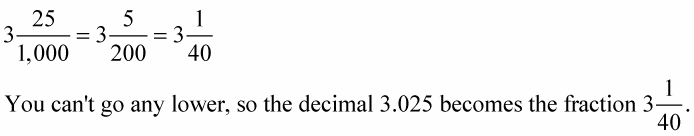Check out the decimal-to-fraction conversion in the following examples:

• .3 has just one digit (3) to the right of the decimal point, so .3 becomes the fraction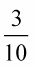• .408 has three digits (408) to the right of the decimal point, so .408 becomes the fraction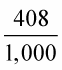• 60.0003 has four digits (0003) to the right of the decimal point, but it also has a number that falls before the decimal, which becomes the whole number of the fraction. So 60.0003 becomes the fraction